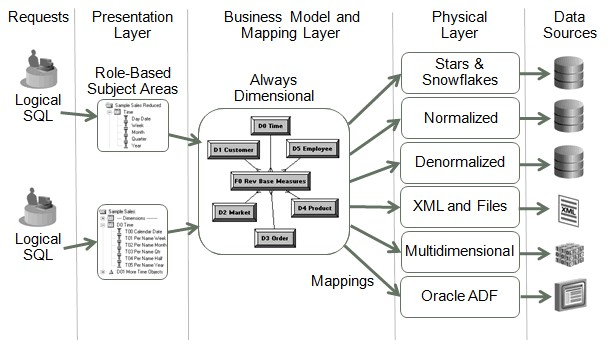# Function - Display Function

A display function is a function that operates on the result set of a query.

They computes their result on a query result set such as:

• ranks,
• Ntiles,
• standard deviations,
• running totals,
• moving averages,
• and moving medians.

Recommended PagesOBIEE - (Display Function|Derived Measures) (Rank, TopN, Median, )

Oracle BI Answers simplifies the use of derived measures i.e. measures that are computed on a query result set such as ranks, Ntiles, standard deviations, running totals, moving averages, and moving medians....Ordinal Data - Rank function (Ranking)

Rank is a statistic / function that calculates the rank of a value in a group of values. The position of an element in a sequence is its rank or index. This function is used in: top-N and bottom-N...